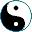More Examples of Functors
<<
>>
 Again, lets take a look at small categories and their functors. If we consider groups as categories, what would be their functors? A functor must preserve unit morphism and composition. Hence, a functor is just a group homomorphism. Any order-preserving (a.k.a. monotonous) function between two partially ordered sets is a functor. Take a pair of oriented graphs and a ma that preserve edges. We can extend this map to a function that maps path from one graph to paths of another graph. This function, by definition, preserves concatenation and empty paths, thus it is a functor from one graph-generated category to another. Remember category 1? Now, how would a functor from 1 to a category C look like? 1 has just one object, and an identity morphism. So, to specify such a functor is the same as to select an object in C - and vice versa, for any object X in category C we can specify a functor PointX: 1 → C